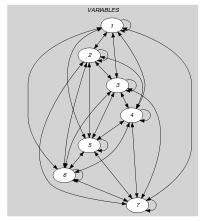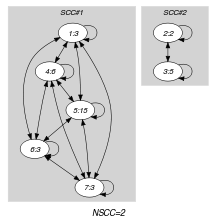## 5.274. nequivalence

Origin

Derived from $\mathrm{𝚗𝚟𝚊𝚕𝚞𝚎}$.

Constraint

$\mathrm{𝚗𝚎𝚚𝚞𝚒𝚟𝚊𝚕𝚎𝚗𝚌𝚎}\left(\mathrm{𝙽𝙴𝚀𝚄𝙸𝚅},𝙼,\mathrm{𝚅𝙰𝚁𝙸𝙰𝙱𝙻𝙴𝚂}\right)$

Arguments
 $\mathrm{𝙽𝙴𝚀𝚄𝙸𝚅}$ $\mathrm{𝚍𝚟𝚊𝚛}$ $𝙼$ $\mathrm{𝚒𝚗𝚝}$ $\mathrm{𝚅𝙰𝚁𝙸𝙰𝙱𝙻𝙴𝚂}$ $\mathrm{𝚌𝚘𝚕𝚕𝚎𝚌𝚝𝚒𝚘𝚗}\left(\mathrm{𝚟𝚊𝚛}-\mathrm{𝚍𝚟𝚊𝚛}\right)$
Restrictions
 $\mathrm{𝚛𝚎𝚚𝚞𝚒𝚛𝚎𝚍}$$\left(\mathrm{𝚅𝙰𝚁𝙸𝙰𝙱𝙻𝙴𝚂},\mathrm{𝚟𝚊𝚛}\right)$ $\mathrm{𝙽𝙴𝚀𝚄𝙸𝚅}\ge \mathrm{𝚖𝚒𝚗}\left(1,|\mathrm{𝚅𝙰𝚁𝙸𝙰𝙱𝙻𝙴𝚂}|\right)$ $\mathrm{𝙽𝙴𝚀𝚄𝙸𝚅}\le \mathrm{𝚖𝚒𝚗}\left(𝙼,|\mathrm{𝚅𝙰𝚁𝙸𝙰𝙱𝙻𝙴𝚂}|\right)$ $\mathrm{𝙽𝙴𝚀𝚄𝙸𝚅}\le$$\mathrm{𝚛𝚊𝚗𝚐𝚎}$$\left(\mathrm{𝚅𝙰𝚁𝙸𝙰𝙱𝙻𝙴𝚂}.\mathrm{𝚟𝚊𝚛}\right)$ $𝙼>0$
Purpose

$\mathrm{𝙽𝙴𝚀𝚄𝙸𝚅}$ is the number of distinct rests obtained by dividing the variables of the collection $\mathrm{𝚅𝙰𝚁𝙸𝙰𝙱𝙻𝙴𝚂}$ by $𝙼$.

Example
$\left(2,3,〈3,2,5,6,15,3,3〉\right)$

Since the expressions $3\mathrm{mod}3=0$, $2\mathrm{mod}3=2$, $5\mathrm{mod}3=2$, $6\mathrm{mod}3=0$, $15\mathrm{mod}3=0$, $3\mathrm{mod}3=0$, and $3\mathrm{mod}3=0$ involve two distinct values (values 0 and 2), the first argument $\mathrm{𝙽𝙴𝚀𝚄𝙸𝚅}$ of the $\mathrm{𝚗𝚎𝚚𝚞𝚒𝚟𝚊𝚕𝚎𝚗𝚌𝚎}$ constraint is set to value 2.

Typical
 $\mathrm{𝙽𝙴𝚀𝚄𝙸𝚅}>1$ $\mathrm{𝙽𝙴𝚀𝚄𝙸𝚅}<|\mathrm{𝚅𝙰𝚁𝙸𝙰𝙱𝙻𝙴𝚂}|$ $\mathrm{𝙽𝙴𝚀𝚄𝙸𝚅}<$$\mathrm{𝚛𝚊𝚗𝚐𝚎}$$\left(\mathrm{𝚅𝙰𝚁𝙸𝙰𝙱𝙻𝙴𝚂}.\mathrm{𝚟𝚊𝚛}\right)$ $𝙼>1$ $𝙼<$$\mathrm{𝚖𝚊𝚡𝚟𝚊𝚕}$$\left(\mathrm{𝚅𝙰𝚁𝙸𝙰𝙱𝙻𝙴𝚂}.\mathrm{𝚟𝚊𝚛}\right)$
Symmetries
• Items of $\mathrm{𝚅𝙰𝚁𝙸𝙰𝙱𝙻𝙴𝚂}$ are permutable.

• An occurrence of a value $u$ of $\mathrm{𝚅𝙰𝚁𝙸𝙰𝙱𝙻𝙴𝚂}.\mathrm{𝚟𝚊𝚛}$ can be replaced by any other value $v$ such that $v$ is congruent to $u$ modulo $𝙼$.

Arg. properties
• Functional dependency: $\mathrm{𝙽𝙴𝚀𝚄𝙸𝚅}$ determined by $𝙼$ and $\mathrm{𝚅𝙰𝚁𝙸𝙰𝙱𝙻𝙴𝚂}$.

• Contractible wrt. $\mathrm{𝚅𝙰𝚁𝙸𝙰𝙱𝙻𝙴𝚂}$ when $\mathrm{𝙽𝙴𝚀𝚄𝙸𝚅}=1$ and $|\mathrm{𝚅𝙰𝚁𝙸𝙰𝙱𝙻𝙴𝚂}|>0$.

• Contractible wrt. $\mathrm{𝚅𝙰𝚁𝙸𝙰𝙱𝙻𝙴𝚂}$ when $\mathrm{𝙽𝙴𝚀𝚄𝙸𝚅}=|\mathrm{𝚅𝙰𝚁𝙸𝙰𝙱𝙻𝙴𝚂}|$.

• Extensible wrt. $\mathrm{𝚅𝙰𝚁𝙸𝙰𝙱𝙻𝙴𝚂}$ when $\mathrm{𝙽𝙴𝚀𝚄𝙸𝚅}=𝙼$.

Algorithm

Since constraints $X=Y$ and $X\equiv Y\left(\mathrm{mod}M\right)$ are similar, one should also use a similar algorithm as the one [Beldiceanu01], [BeldiceanuCarlssonThiel02] provided for constraint $\mathrm{𝚗𝚟𝚊𝚕𝚞𝚎}$.

related: $\mathrm{𝚗𝚌𝚕𝚊𝚜𝚜}$ ($\mathrm{𝚟𝚊𝚛𝚒𝚊𝚋𝚕𝚎}\mathrm{mod}\mathrm{𝚌𝚘𝚗𝚜𝚝𝚊𝚗𝚝}$ replaced by $\mathrm{𝚟𝚊𝚛𝚒𝚊𝚋𝚕𝚎}\in \mathrm{𝚙𝚊𝚛𝚝𝚒𝚝𝚒𝚘𝚗}$), $\mathrm{𝚗𝚒𝚗𝚝𝚎𝚛𝚟𝚊𝚕}$ ($\mathrm{𝚟𝚊𝚛𝚒𝚊𝚋𝚕𝚎}\mathrm{mod}\mathrm{𝚌𝚘𝚗𝚜𝚝𝚊𝚗𝚝}$ replaced by $\mathrm{𝚟𝚊𝚛𝚒𝚊𝚋𝚕𝚎}/\mathrm{𝚌𝚘𝚗𝚜𝚝𝚊𝚗𝚝}$), $\mathrm{𝚗𝚙𝚊𝚒𝚛}$ ($\mathrm{𝚟𝚊𝚛𝚒𝚊𝚋𝚕𝚎}\mathrm{mod}\mathrm{𝚌𝚘𝚗𝚜𝚝𝚊𝚗𝚝}$ replaced by $\mathrm{𝚙𝚊𝚒𝚛}$ of $\mathrm{𝚟𝚊𝚛𝚒𝚊𝚋𝚕𝚎𝚜}$).

specialisation: $\mathrm{𝚗𝚟𝚊𝚕𝚞𝚎}$ ($\mathrm{𝚟𝚊𝚛𝚒𝚊𝚋𝚕𝚎}\mathrm{mod}\mathrm{𝚌𝚘𝚗𝚜𝚝𝚊𝚗𝚝}$ replaced by $\mathrm{𝚟𝚊𝚛𝚒𝚊𝚋𝚕𝚎}$).

Keywords
Arc input(s)

$\mathrm{𝚅𝙰𝚁𝙸𝙰𝙱𝙻𝙴𝚂}$

Arc generator
$\mathrm{𝐶𝐿𝐼𝑄𝑈𝐸}$$↦\mathrm{𝚌𝚘𝚕𝚕𝚎𝚌𝚝𝚒𝚘𝚗}\left(\mathrm{𝚟𝚊𝚛𝚒𝚊𝚋𝚕𝚎𝚜}\mathtt{1},\mathrm{𝚟𝚊𝚛𝚒𝚊𝚋𝚕𝚎𝚜}\mathtt{2}\right)$

Arc arity
Arc constraint(s)
$\mathrm{𝚟𝚊𝚛𝚒𝚊𝚋𝚕𝚎𝚜}\mathtt{1}.\mathrm{𝚟𝚊𝚛}\mathrm{mod}𝙼=\mathrm{𝚟𝚊𝚛𝚒𝚊𝚋𝚕𝚎𝚜}\mathtt{2}.\mathrm{𝚟𝚊𝚛}\mathrm{mod}𝙼$
Graph property(ies)
$\mathrm{𝐍𝐒𝐂𝐂}$$=\mathrm{𝙽𝙴𝚀𝚄𝙸𝚅}$

Graph model

Parts (A) and (B) of Figure 5.274.1 respectively show the initial and final graph associated with the Example slot. Since we use the $\mathrm{𝐍𝐒𝐂𝐂}$ graph property we show the different strongly connected components of the final graph. Each strongly connected component corresponds to one equivalence class: We have two equivalence classes that respectively correspond to values $\left\{3,6,15\right\}$ and $\left\{2,5\right\}$.

##### Figure 5.274.1. Initial and final graph of the $\mathrm{𝚗𝚎𝚚𝚞𝚒𝚟𝚊𝚕𝚎𝚗𝚌𝚎}$ constraint(a) (b)